voltage. In a parallel circuit the source current divides among the available paths."> Current in a Parallel CircuitCustom Search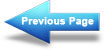Voltage in a Parallel Circuit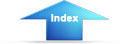Matter, Energy, and ElectricityResistance in a Parallel CircuitCurrent in a Parallel Circuit

Ohm's law states that the current in a circuit is inversely proportional to the circuit resistance. This fact is true in both series and parallel circuits.

There is a single path for current in a series circuit. The amount of current is determined by the total resistance of the circuit and the applied voltage. In a parallel circuit the source current divides among the available paths.

The behavior of current in parallel circuits will be shown by a series of illustrations using example circuits with different values of resistance for a given value of applied voltage.

Part (A) of figure 3-40 shows a basic series circuit. Here, the total current must pass through the single resistor. The amount of current can be determined.

Figure 3-40. - Analysis of current in parallel circuit.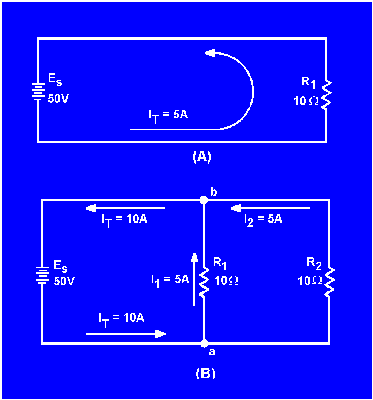Given:Solution: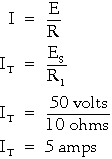Part (B) of figure 3-40 shows the same resistor (R1) with a second resistor (R2) of equal value connected in parallel across the voltage source. When Ohm's law is applied, the current flow through each resistor is found to be the same as the current through the single resistor in part (A).

Given: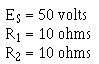Solution:It is apparent that if there is 5 amperes of current through each of the two resistors, there must be a TOTAL CURRENT of 10 amperes drawn from the source.

The total current of 10 amperes, as illustrated in figure 3-40(B leaves the negative terminal of the battery and flows to point a. Since point a is a connecting point for the two resistors, it is called a JUNCTION. At junction a, the total current divides into two currents of 5 amperes each. These two currents flow through their respective resistors and rejoin at junction b. The total current then flows from junction b back to the positive terminal of the source. The source supplies a total current of 10 amperes and each of the two equal resistors carries one-half the total current.

Each individual current path in the circuit of figure 3-40(B) is referred to as a BRANCH. Each branch carries a current that is a portion of the total current. Two or more branches form a NETWORK.

From the previous explanation, the characteristics of current in a parallel circuit can be expressed in terms of the following general equation:

IT = I1 + I2 + . . . In

Compare part (A) of figure 3-41 with part (B) of the circuit in figure 3-40. Notice that doubling the value of the second branch resistor (R2) has no effect on the current in the first branch (IR1), but does reduce the second branch current (IR2) to one-half its original value. The total circuit current drops to a value equal to the sum of the branch currents. These facts are verified by the following equations.

Given: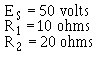Solution:Figure 3-41. - Current behavior in parallel circuits.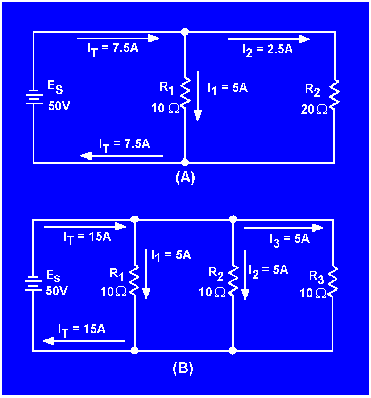The amount of current flow in the branch circuits and the total current in the circuit shown in figure 3-41(B) are determined by the following computations.

Given: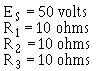Solution: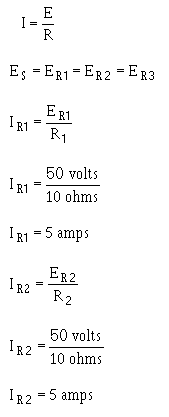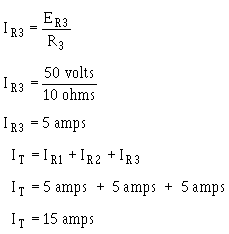Notice that the sum of the ohmic values in each circuit shown in figure 3-41 is equal (30 ohms), and that the applied voltage is the same (50 volts). However, the total current in 3-41(B) (15 amps) is twice the amount in 3-41(A) (7.5 amps). It is apparent, therefore, that the manner in which resistors are connected in a circuit, as well as their actual ohmic values, affect the total current.

The division of current in a parallel network follows a definite pattern. This pattern is described by KIRCHHOFF'S CURRENT LAW which states:

"The algebraic sum of the currents entering and leaving any junction of conductors is equal to zero."

This law can be stated mathematically as:

Ia + Ib + . . . In + 0

where: Ia, Ib, etc., are the currents entering and leaving the junction. Currents ENTERING the junction are considered to be POSITIVE and currents LEAVING the junction are considered to be NEGATIVE. When solving a problem using Kirchhoff's current law, the currents must be placed into the equation WITH THE PROPER POLARITY SIGNS ATTACHED.

Example. Solve for the value of I3 in figure 3-42.

Given: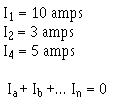Solution:

Ia + Ib + . . . I
a
+ 0

Figure 3-42. - Circuit for example problem.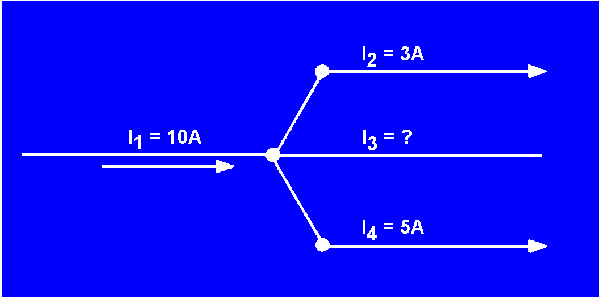The currents are placed into the equation with the proper signs.I3 has a value of 2 amperes, and the negative sign shows it to be a current LEAVING the junction.

Example. Using figure 3-43, solve for the magnitude and direction of I3.

Figure 3-43. - Circuit for example problem.Given:Solution: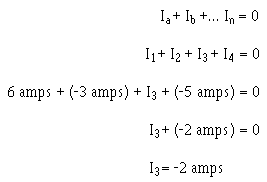I3 is 2 amperes and its positive sign shows it to be a current entering the junction.

There is a relationship between total current and current through the individual components in a circuit. What is this relationship in a series circuit and a parallel circuit?

In applying Kirchhoff's current law, what does the polarity of the current indicate?Integrated Publishing, Inc. - A (SDVOSB) Service Disabled Veteran Owned Small Business